# GMAT Quant: Geometry – Perimeter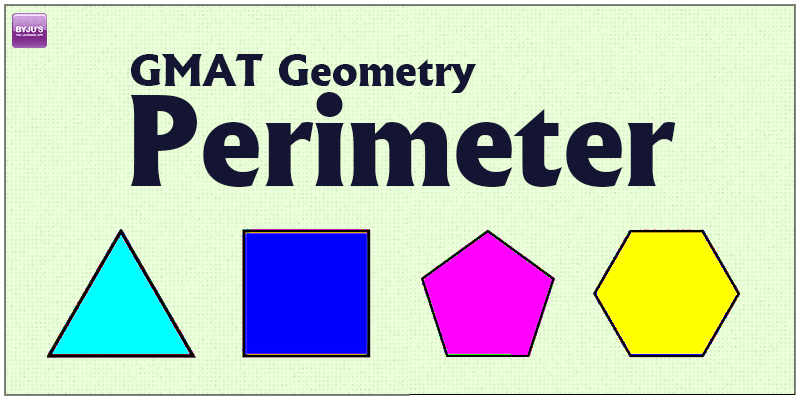You’re bound to get question on Perimeter in GMAT Quant. Geometry is all about figures and their formulas. In our previous post, we have jot down all GMAT Quant Geometry Formulas for your convenience. Here is a detailed discussion on Perimeter and it’s application.

What is a Perimeter?

Perimeter, it is the complete length of the lines forming the outline of any shape. To calculate the perimeter of any shape, add up all the sides of the shape. All standard shapes have a fixed perimeter formula and if the shapes are not a regular one, then you can divide it to a regular shape and then calculate it.

Let’s see some standard shapes and formulas:

1. Circle: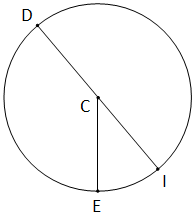Perimeter of a circle is equal to

Where, r = radius and d = diameter.

1. Perimeter of square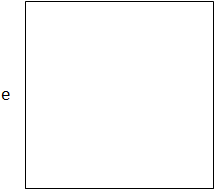Perimeter = 4e

1. Overall you simply add all the sides of a shape and you can get the perimeter of that shape.

Let’s solve a question to get a better idea of the type of question GMAT asks.

Question: A rectangular switch board is made such that its length is ‘l’ inches and perimeter is ‘p’ inches. If it has an area of ‘a’ sq. inches, which of the following is true?

1. l2 + pl + a = 0
2. l2 – pl + 2a = 0
3. 2l2 + pl + 2a = 0
4. 2l2 – pl – 2a = 0
5. 2l2 – pl + 2a = 0

Solution:

Perimeter = 2 (l + b)

P = 2 (l + b)

b =p/2-l

l (p/2-l)=a

Area is given as ‘a’

lb = a

lp – 2l2 = 2a

2l2 – pl + 2a = 0

Question: Calculate the perimeter of the parallelogram: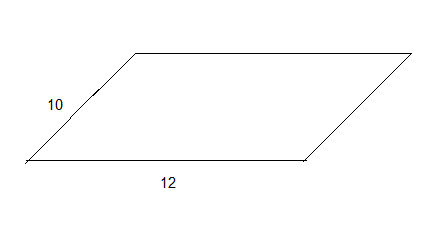Solution: In a parallelogram, the opposite sides are equal in length. Hence, the figure becomes: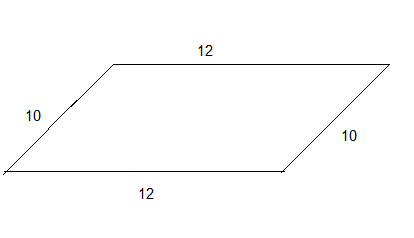So, the perimeter becomes: 2= 44

Question: Calculate the perimeter of the given figure: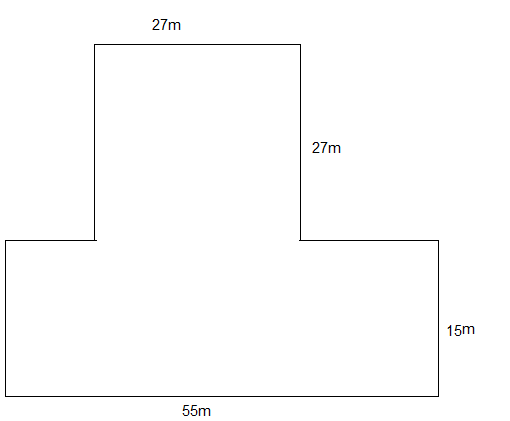Solution: This figure can be divided into two figures: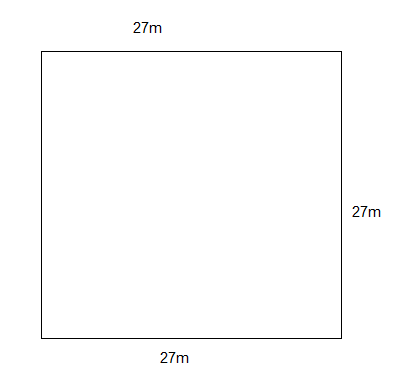Perimeter = 4

And,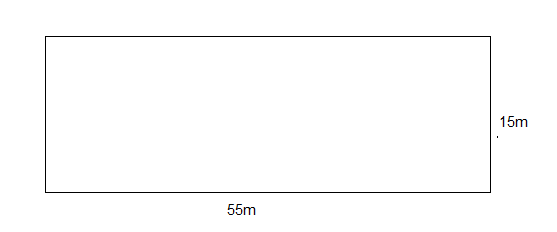Perimeter = 2 (15 + 55)

= 2

So, overall perimeter = (108 + 140) – 227 = 194m

To learn more tricks on perimeter, give us a missed call or drop a SMS at +918884544444. You can write to us at: byjusforgmat@gmail,com